#### Wavefront propagation algorithms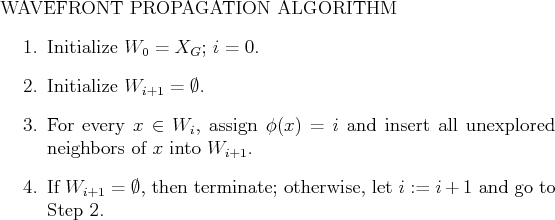Once the approximation has been made, any of the methods discussed in Section 8.2.2 can be used to compute a navigation function. An optimal navigation function can be easily computed using Dijkstra's algorithm from the goal. If each motion has unit cost, then a useful simplification can be made. Figure 8.4 describes a wavefront propagation algorithm that computes an optimal navigation function. It can be considered as a special case of Dijkstra's algorithm that avoids explicit construction of the priority queue. In Dijkstra's algorithm, the cost of the smallest element in the queue is monotonically nondecreasing during the execution of the algorithm. In the case of each motion having unit cost, there will be many states in the queue that have the same cost. Dijkstra's algorithm could remove in parallel all elements that have the same, smallest cost. Suppose the common, smallest cost value is. These states are organized into a wavefront,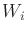. The initial wavefront is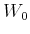, which represents the states in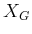. The algorithm can immediately assign an optimal cost-to-go value ofto every state that can be reached in one stage from any state in. These must be optimal because no other cost value is optimal. The states that receive costcan be organized into the wavefront. The unexplored neighbors ofare assigned cost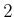, which also must be optimal. This process repeats inductively fromto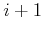until all reachable states have been reached. In the end, the optimal cost-to-go is computed in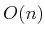time, in whichis the number of reachable grid states. For any states that were not reached, the value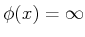can be assigned. The navigation function shown in Figure 8.3 can actually be computed using the wavefront propagation algorithm.

Steven M LaValle 2020-08-14